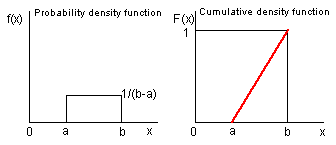### Why should I choose AnalystNotes?

AnalystNotes specializes in helping candidates pass. Period.

##### Subject 4. The Discrete Uniform Distribution
A uniform distribution is one for which the probability of occurrence is the same for all values of X. It is just one type of special random variable and is sometimes called a rectangular distribution. For example, if a die is thrown, the probability of obtaining any one of the six possible outcomes is 1/6. Since all outcomes are equally probable, the distribution is uniform. If a uniform distribution is divided into equally spaced intervals, there will be an equal number of members of the population in each interval.

The discrete uniform distribution is the simplest of all probability distributions. This distribution has a finite number of specified outcomes, and each outcome is equally likely. Mathematically, suppose that a discrete uniform random variable, X, has n possible outcomes: x1, x2, ..., xn-1, and xn.

• p(x1) = p(x2) = p(x3) = ... = p(xn-1) = p(xn) = p(x). That is, the probabilities for all possible outcomes are equal.
• F(xk) = kp(xk). That is, the cumulative distribution function for the kth outcome is k times of the probability of the kth outcome.
• If there are k possible outcomes in a particular range, the probability for that range of outcomes is kp(X).

For example, the possible outcomes are the integers 1 to 8 (inclusive), and the probability that the random variable takes on any of those possible values is the same for all outcomes (i.e., it is uniform).

If a continuous random variable is equally likely to fall at any point between its maximum and minimum values, it is a continuous uniform random variable, and its probability distribution is a continuous probability distribution.

• The probability density function is: f(x) = 1/(b - a) for a ≤ x ≤ b; or 0 otherwise.
• The cumulative density function is: F(x) = 0 for x ≤ a; (x - a)/(b - a) for a ≤ x ≤ b; 1 for x ≥ b.• The probability density function is a horizontal line with a height of 1/(b-a) over a range of values from a to b.
• The cumulative density function is a sloped line with a height of 0 to 1 over a range of values from a to b, and is a horizontal line with a height of 1 when the value of the variable equals or exceeds b.

For example, with a = 0 and b = 8, f(x) = 0.125. If this density is graphed, it will plot as a horizontal line with a value of 0.125.

To find the probability F(3) = P(X ≤ 3), find the area under the curve graphing the probability density function, between 0 and 3 on the x-axis. The middle line of the expression for the cumulative probability function is:

F(x) = 0 for x ≤ a; (x - a)/(b - a) for a < x < b; 1 for x ≥ b

For a continuous uniform random variable, the mean is given by μ = (a + b)/2 and the variance is given by σ2 = (b - a)2/12.

Learning Outcome Statements

e. define a discrete uniform random variable, a Bernoulli random variable, and a binomial random variable;

f. calculate and interpret probabilities given the discrete uniform and the binomial distribution functions;

g. construct a binomial tree to describe stock price movement;

h. calculate and interpret tracking error;

i. define the continuous uniform distribution and calculate and interpret probabilities, given a continuous uniform distribution;

CFA® 2021 Level I Curriculum, , Volume 1, Reading 9

User Comment
got2pass why is the variance for the continuous uniform random variable divisible by 12?
markh that's the definition. check any stats textbook.
apiccion No, it's not by definition.

You can work it out algebraically from the definition of variance. You will need to know the formula for the sum of squares and sum of the counting numbers: http://www.cs.ecu.edu/~hochberg/fall2002/class10/fslide1.html

Notice the denominators of the two formulas when multiplied together give 12.
tschorsch you can move the distribution up or down and the variance will be the same

assume L = b-a

take the interval -L/2 to L/2 instead

the variance is the average of the (x-mean(x))²
since in our case mean(x) is 0
the variance average is just value of x²
over the interval -L/2 to L/2

the average is the same above and below x=0
so the area from 0 to L/2 is ((L/2)³ /3)
or L³/24
by 2 for -L/2 to 0
so the area is L³/12

divide by L to get the average

you get L²/12

but L is just b-a (i.e. the length)

so (b-a)²/12 is the variance

note: the area under x² is calculated by the integrating x² which is x³/3, call this G(x)

the area from 0 to L/2 is G(L/2) - G(0)
AUAU I know the formula for prob should be born in mind. Does anyone know we should memorise the variance?
Allen88 If you type into google, variance formula for continuous uniform distribution derivation, click on, statistics for business and financial economics-google result, scroll down a little and you'll see the derivation for both the mean and variance.
dethepp1 For the cumulative density function: How is it possible, that the result for x = a and x = b is defined twice. In my eyes that's a mistake in the notes, but could someone submit my suggestion. It should state F(x) for a > x the result is zero and b < x the value of F(x) is 1......